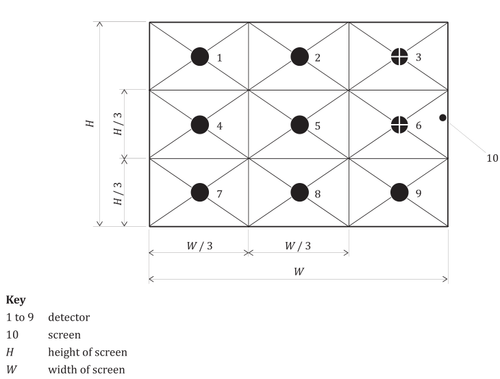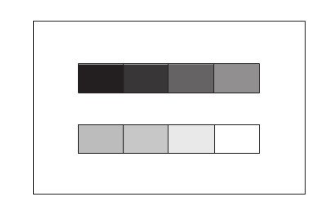### A1. Luminance Test Conditions

1. Test environment: Dark room at a temperature between 15 ℃ and 25℃ and relative humidity between 30 %RH and 70 %RH.
2. The cumulative use time of the testing product is less than 10 hours.
3. While testing, the power supply needs to be plugged in to the testing product (including the testing product with a rechargeable battery).
4. The testing product is set to the brightest mode.
5. Well-focused 80 inches of projection area.
6. Input a greyscale test pattern image (as shown in Figure 2 in Appendix A2) to the testing product. Adjusting the brightness and contrast ratio until the difference of gray patches between the extreme black and white are recognizable by human eyes.
7. Error between the measured value and nominal value ≤ 20%.
8. Input the test feature image: 100% full-white pattern image (as shown in Figure 1 in Appendix A2).
9. Calculation method Use illumination photometer to measure the brightness of the center point in 9 boxes showed in the below Figure A1 nine-point grid, and use the following formula to calculate the brightness value of light output. Brightness value of light output I：= Average (L1, L2 … L9)*S S: Projection area (length × height), Unit: square meters (m²)
10. Expression of measurement results The measurement results are expressed in lumens (ANSI lm).

### The example of the lumen test nine-point grid is shown in Figure 1.Figure 1 - 9 points measuring grid

Greyscale test pattern is shown in Figure 2Figure 2 - Greyscale test pattern

Appendix:
Test standard reference: "GB 32028-2015","ISO/IEC 21118: 2020(E)"